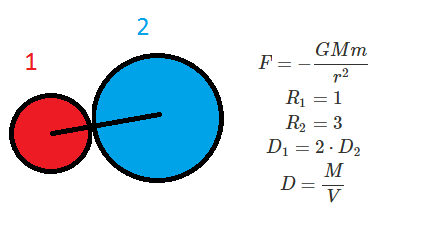# The Effect of Scaling on Gravitational ForceTwo solid metal balls are placed in contact with each other. The first ball is made of a material twice as dense as the second ball; the first ball has a radius of 1, the second has a radius of 3. The gravitational force between them is $F_1$. The balls are somehow enlarged so that they gain mass proportional to their respective densities (density is conserved) and ball one's radius is twice as large as it was and ball two's radius is five times as large as it was. What is the new force relative to $F_1$?

×

Problem Loading...

Note Loading...

Set Loading...